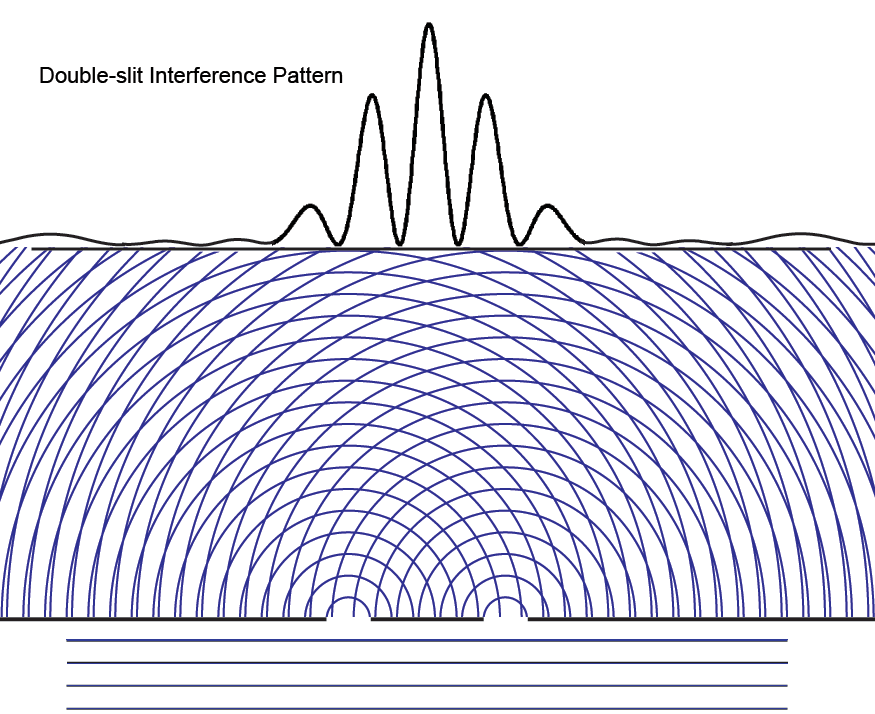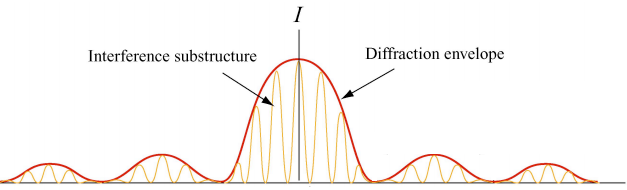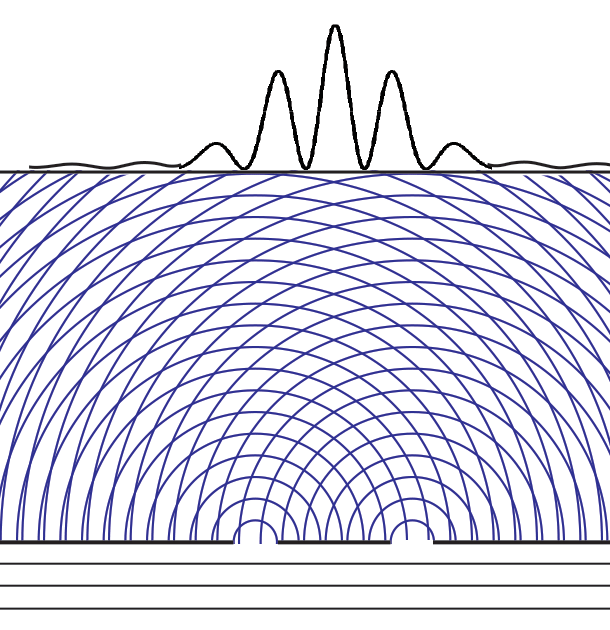Collapse of the Wave Function

Why is it that more than half of the modern "interpretations" of quantum mechanics deny the "collapse of the wave function?," also known as Paul Dirac's "projection postulate."

Why are so many serious physicists and philosophers of science so unhappy with this concept, which is a fundamental part of the "orthodox" theory proposed in the late 1920's by the "founders" of quantum mechanics - Werner Heisenberg, Niels Bohr, Max Born, Wolfgang Pauli, and Pascual Jordan, and Dirac.

We can give the simplest answer in a single word - chance. Albert Einstein, the foremost scientist of all time adamantly disliked the idea of "uncertainty" or "indeterminism," the thought that some things in the universe were not caused (or only statistically caused). Ironically, Einstein was the discoverer of chance in quantum mechanics, which he disliked but never denied that indeterminism was a part of a statistical quantum theory, as far as quantum theory could go in his time.

The idea of the wave function in quantum mechanics and its indeterministic collapse during a measurement is without a doubt among the three most controversial problems in quantum physics today, the others being the two-slit experiment and entanglement..

So it is very important to understand the importance of what Dirac called the projection postulate in quantum mechanics. The “collapse of the wave function” is also known as the “reduction of the wave packet.” This describes the change from a system that can be seen as having many possible quantum states (Dirac’s principle of superposition) to its randomly being found in only one of those possible states.

Although the collapse is historically thought to be caused by a measurement, and thus dependent on the role of the observer in preparing and running the experiment, collapses can occur whenever quantum systems interact (e.g., collisions between particles) or even spontaneously (e.g., in the decay of radioactive nuclei).

The claim that an observer is needed to collapse the wave function has injected a severely anthropomorphic element into quantum theory, suggesting that nothing happens in the universe except when physicists are making measurements. An extreme example is Hugh Everett’s Many Worlds theory, which says that the universe splits into two nearly identical universes whenever a physical measurement is made.

What is the Wave Function?
As Einstein’s blackboard drawing at the Solvay Conference shows us, the wave function propagates like a light wave, but when the particle appears, it is found at a single point P.Using Einstein’s idea of “objective reality,” without any interactions that could change the momentum, the particle must have traveled in a straight line from the origin to the point where it is found.

Einstein tells us the wave represents the probability of finding the particle. (Today it is the absolute square of the complex wave function Ψ that gives us the probability.) All directions are equally probable until the moment when the particle is found somewhere. At that moment, the probability of its being elsewhere goes to zero.

This has been interpreted as a “collapse.” If the wave had been carrying energy in all directions, or matter as Schrödinger thought, energy and matter would indeed have had to “collapse” to the point.

Perhaps the best illustration of the wave function is to show it passing though the famous slits in a two-slit experiment. It has been known for centuries that water waves passing through a small opening create circular waves radiating outward from that opening. If there are two openings, the waves from each opening interfere with those from the other, producing waves twice as high in the crests (or twice as deep in the troughs) and cancelling perfectly where a crest from one meets a trough from the other.

When we send light through tiny slits, we see a similar wave phenomenon. Despite the standard description, and although light energy is obviously moving, we will show that the wave is not moving and does not really "collapse." In the water example, there is actually very little, if any, water moving forward. A cork bobs up and down as waves pass it but water does not not pass the cork. The wave function is the solution of the Schrödinger equation, given the wavelength of the particle and the boundary conditions of the experiment.

We can regard it as a standing wave, with locations for null points (where the probability of finding a particle is zero) fixed at locations on the detection screen.Most of the light that reaches light detectors in the screen at the back lands right behind the barrier between the two slits. At some places, no light appears in the interference pattern.

If we look closely, the interference pattern is a kind of substructure inside an envelope that corresponds to the Fraunhofer diffraction pattern if the two slits were replaced by one large slit.Today, thanks to the perseverance of Einstein, we know that light actually consists of large numbers of individual photons, quanta of light. Our experiment can turn down the amount of light so low that we know there is only a single photon, a single particle of light in the experiment at any time. What we see is the very slow accumulation of photons at the detectors, but with exactly the same interference pattern when a large number of photons has been detected. And this leads to what Richard Feynman called not just "a mystery,” but actually "the only mystery” in quantum mechanics.

How can a single particle of light interfere with itself, without going through both slits? We can see what would happen if it went through only one slit by closing one of them. We get a completely different interference pattern. Information philosophy now lets us visualize this highly non-intuitive phenomenon, one that Dirac says is impossible in classical physics. If you can follow, you are well on your way to comprehending, though perhaps not fully “understanding," quantum mechanics.From the time I first taught my students about the two-slit experiment in the late 1950's, I have always said that "something must be moving through both slits." But I am now questioning the idea that the wave function must be moving to explain the "collapse." Has the choice of words muddied the waters, so to speak? Let's start with the view that the wave function moves.

The wave function in quantum mechanics is a solution to Erwin Schrödinger’s famous wave equation that describes the evolution in time of his wave function ψ,

ih/2π δψ/δt = Hψ.

Following suggestions by Einstein about light waves and light particles in the years before 1920, Max Born in 1926 interpreted the wave function ψ(x) for a material particle at a position x as telling us that the complex square of the wave function, < ψ(x) | ψ(x) >, gives us the probability of finding a particle at that position. He was generalizing Einstein's radical idea, from nearly ten years earlier, that a light wave's amplitude (its energy) at a point is a measure of finding a light quantum there.

Compare David Bohm's "pilot wave" that enters both slits, where the particle being carried along by the wave enters only one. The wave interferes with itself, creating the interference
So the quantum wave function going through the slits (and this probability amplitude wave ψ(x) does go through both slits) is an abstract number, neither material nor energy, just a probability. It is information about where particles of light (or particles of matter if we shoot electrons at the slits) will be found when we record a large number of them.

If we imagine a single particle being sent from a great distance away toward the two slits, the wave function that describes its “time evolution” or motion through space looks like a plane wave - the straight lines of the wave crests approaching the slits from below in the figure to the left. We have no information about the exact position of the particle. It could be anywhere. Einstein said that the Copenhagen interpretation of quantum mechanics is “incomplete” because the particle has no definite position before a measurement. He was right. When the particle lands on one of the detectors at the screen in back, we can represent it by the dot in the figure below.

Animation of a wave function collapsing - click to restartThe interfering probability amplitude waves disappear instantly everywhere once the particle is detected, but we have left a small fragment of interfering waves in the upper left corner to ask a question first seen by Einstein in 1905, then made explicit at the 1927 Solvay Conference..

What happens to the small but finite probability that the particle might have been found at the left side of the screen? How has that probability instantaneously (at faster than light speed) been collected into the unit probability at the dot?

To be clear, when Einstein first asked this question, he thought of the light wave as energy spread out everywhere in the wave. So it was energy that he thought might be traveling faster than light, violating his brand new principle of relativity (published two months after his light quantum paper).

At the Solvay conference in Brussels in 1927, twenty-two years after Einstein first tried to understand what is happening when the wave collapses, he noted;

If | ψ |2 were simply regarded as the probability that at a certain point a given particle is found at a given time, it could happen that the same elementary process produces an action in two or several places on the screen. But the interpretation, according to which | ψ |2 expresses the probability that this particle is found at a given point, assumes an entirely peculiar mechanism of action at a distance, which prevents the wave continuously distributed in space from producing an action in two places on the screen.”

Einstein came to call this spukhafte Fernwerkung, “spooky action at a distance.” It is known as nonlocality.

Niels Bohr recalled Einstein’s description. He drew Einstein's figure on a blackboard, but he did not understand what Einstein was saying.

Einstein referred at one of the sessions to the simple example, illustrated by Fig. 1, of a particle (electron or photon) penetrating through a hole or a narrow slit in a diaphragm placed at some distance before a photographic plate.On account of the diffraction of the wave connected with the motion of the particle and indicated in the figure by the thin lines, it is under such conditions not possible to predict with certainty at what point the electron will arrive at the photographic plate, but only to calculate the probability that, in an experiment, the electron will be found within any given region of the plate.

The apparent difficulty, in this description, which Einstein felt so acutely, is the fact that, if in the experiment the electron is recorded at one point A of the plate, then it is out of the question of ever observing an effect of this electron at another point (B), although the laws of ordinary wave propagation offer no room for a correlation between two such events.

Bohr's point A is the dot in our animation where the particle is found. His point B is Einstein's concern about another point where there is/was a non-zero probability of finding the particle.
How Information Physics Explains the Two-Slit Experiment
Although we cannot say anything about the particle’s whereabouts, we can say clearly that what goes through the two slits and interferes with itself is information. The wave function tells us the abstract probability of finding the particle somewhere.

In our latest information interpretation of quantum mechanics, we ask where the information about the probabilities of finding a particle are really coming from?With one slit open, there is a broad Fraunhofer pattern of interference when the slit width is the about the same as the light wavelength. Those who say there is no interference with only one slit open do not understand this.Opening a second slit, there are more interference minima and now the maximum is behind the wall between the slits. Some places where there was radiation detected now are null points! Any light coming in the second slit appears to be cancelling the light from the first slit!

Note that probability, like information, is neither matter nor energy. When a wave function “collapses” or “goes through both slits” in the dazzling two-slit experiment, nothing material is traveling faster than the speed of light or going through the slits. No messages or signals can be sent using this collapse of probability. Only the information has changed.

This is similar to the Einstein-Podolsky-Rosen experiments, where measurement of one particle transmits nothing physical (matter or energy) to the other “entangled” particle. Instead instantaneous information has come into the universe at the new particle positions. That information, together with conservation of angular momentum, makes the state of the coherently entangled second particle certain, however far away it might be after the measurement.

The standard “orthodox” interpretation of quantum mechanics includes the projection postulate. This is the idea that once one of the possibilities becomes actual at one position, the probabilities for actualization at all other positions becomes instantly zero. New information has appeared.

The principle of superposition tells us that before a measurement, a system may be in any one of many possible states. In the two-slit experiment, this includes all the possible positions where |ψ(x)|2 is not zero. Once the quantum system (the photon or electron) interacts with a specific detector at the screen, all other possibilities vanish. It is perhaps unfortunate that the word “collapse” was chosen, since it suggests some great physical motion.

Just as in philosophy, where the language used can be the source of confusion, we find that thinking about the information involved clarifies the problem.

Normal | Teacher | Scholar